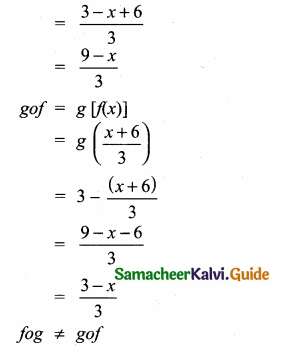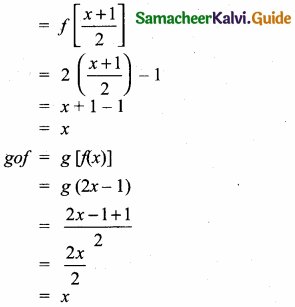Students can download Maths Chapter 1 Relations and Functions Ex 1.5 Questions and Answers, Notes, Samacheer Kalvi 10th Maths Guide Pdf helps you to revise the complete Tamilnadu State Board New Syllabus, helps students complete homework assignments and to score high marks in board exams.

## Tamilnadu Samacheer Kalvi 10th Maths Solutions Chapter 1 Relations and Functions Ex 1.5

Question 1.
Using the functions f and g given below, find fog and gof Check whether fog = gof.

(i) f(x) = x – 6, g(x) = x2
f(x) = x – 6, g(x) = x2
fog = fog (x)
= f(g(x))
fog = f(x)2
= x2 – 6
gof = go f(x)
= g(x – 6)
= (x – 6)2
= x2 – 12x + 36
fog ≠ gof(ii) f(x) = $$\frac { 2 }{ x }$$, g(x) = 2x2 – 1
f(x) – $$\frac { 2 }{ x }$$; g(x) = 2x2 – 1
fag = f[g (x)]
= f(2x2 – 1)
= $$\frac{2}{2 x^{2}-1}$$
gof = g [f(x)]
= g ($$\frac { 2 }{ x }$$)
= 2 ($$\frac { 2 }{ x }$$)2 – 1
$$=2 \times \frac{4}{x^{2}}-1$$
$$=\frac{8}{x^{2}}-1$$
fog ≠ gof

(iii) f(x) = $$\frac { x+6 }{ 3 }$$, g(x) = 3 – x
f(x) = $$\frac { x+6 }{ x }$$, g(x) = 3 – x
fog = f[g(x)]
= f(3 – x)(iv) f(x) = 3 + x, g(x) = x – 4
f(x) = 3 + x ;g(x) = x – 4
fog = f[g(x)]
= f(x – 4)
= 3 + x – 4
= x – 1
gof = g[f(x)]
= g(3 + x)
= 3 + x – 4
= x – 1
fog = gof

(v) f(x) = 4x2 – 1,g(x) = 1 + x
f(x) = 4x2 – 1 ; g(x) = 1 + x
fog = f[g(x)]
= 4(1 + x)
= 4(1 + x)2 – 1
= 4[1 + x2 + 2x] – 1
= 4 + 4x2 + 8x – 1
= 4x2 + 8x + 3
gof = g [f(x)]
= g (4x2 – 1)
= 1 + 4x2 – 1
= 4x2
fog ≠ gof

Question 2.
Find the value of k, such that fog = gof
(i) f(x) = 3x + 2, g(x) = 6x – k
(ii) f(x) = 2x – k, g(x) = 4x + 5
Solution:
(i) f(x) = 3x + 2, g(x) = 6x – k
fog(x) = f(g(x)) = f(6x – k) = 3(6x – k) + 2
= 18x – 3k + 2 …………… (1)
gof(x) = g(f(x)) = g(3x + 2) = 6(3x + 2) – k
= 18x + 12 – k ……………. (2)
(1) = (2)
⇒ 18x – 3k + 2 = 18x + 12 – k
2k = -10
k = -5

(ii) f(x) = 2x – k, g(x) = 4x + 5
fog(x) = f(g(x)) = f(4x + 5) = 2(4x + 5) – k
= 8x + 10 – k ……………… (1)
gof(x) = g(f(x)) = g(2x – k) = 4(2x – k) + 5
= 8x – 4k + 5 ……………. (2)
(1) = (2)
⇒ 8x + 10 – k = 8x – 4k + 5
3k = -5
k = $$\frac{-5}{3}$$

Question 3.
If f(x) = 2x – 1, g(x) = $$\frac { x+1 }{ 2 }$$, show that f o g = g o f = x
f(x) = 2x – 1 ; g(x) = $$\frac { x+1 }{ 2 }$$
fog = f[g(x)]∴ fog = gof = x
Hence it is proved.Question 4.
(i) If f (x) = x2 – 1, g(x) = x – 2 find a, if gof(a) = 1.
(ii) Find k, if f(k) = 2k – 1 and fof (k) = 5.
Solution:
(i) f(x) = x2 – 1, g(x) = x – 2
Given gof(a) = 1
gof(x) = g(f(x)
= g(x2 – 1) = x2 – 1 – 2
= x2 – 3
gof(a) ⇒ a2 – 3 = 1 =+ a2 = 4
a = ± 2
(ii) f(k) = 2k – 1
fo f(k) = 5
f(f(k)m = f(2k – 1) = 5
⇒ 2(2k – 1) – 1 = 5
4 k – 2 – 1 = 5 ⇒ 4k = 8
k = 2Question 5.
Let A,B,C N and a function f: A → B be defined by f(x) = 2x + 1 and g: B → C be defined by g(x) = x2 . Find the range of fog and gof.
f(x) = 2x + 1 ; g(x) = x2
fog = f[g(x)]
= f(x2)
= 2x2 + 1
2x2 + 1 ∈ N
g o f = g [f(x)]
= g (2x + 1)
g o f = (2x + 1)2
(2x + 1)2 ∈ N
Range = {y/y = 2x2 + 1, x ∈ N};
{y/y = (2x + 1)2, x ∈ N)

Question 6.
If f(x) = x2 – 1. Find (i)f(x) = x2 – 1, (ii)fofof
Solution:
(i) f(x) = x2 – 1
fof(x) = f(fx)) = f(x2 – 1)
= (x2 – 1 )2 – 1;
= x4 – 2x2 + 1 – 1
= x4 – 2x2
(ii) fofof = f o f(f(x))
= f o f (x4 – 2x2)
= f(f(x4 – 2x2))
= (x4 – 2x2)2 – 1
= x8 – 4x6 + 4x4 – 1Question 7.
If f : R → R and g : R → R are defined by f(x) = x5 and g(x) = x4 then check if f, g are one – one and fog is one – one?
f(x) = x5 – It is one – one function
g(x) = x4 – It is one – one function
fog = f[g(x)]
= f(x4)
= (x4)5
fag = x20
It is also one-one function.

Question 8.
Consider the functions f(x), g(x), h(x) as given below. Show that (fog)oh = fo(goh) in each case.
(i) f(x) = x – 1, g(x) = 3x + 1 and h(x) = x2
(ii) f(x) = x2, g(x) = 2x and h(x) = x + 4
(iii) f(x) = x – 4, g(x) = x2 and h(x) = 3x – 5
Solution:
(i) f(x) = x – 1, g(x) = 3x + 1 and h(x) = x2
f(x) = x – 1
g(x) = 3x + 1
f(x) = x2
(fog)oh = fo(goh)
LHS = (fog)oh
fog = f(g(x)) = f(3x + 1) = 3x + 1 – 1 = 3x
(fog)oh = (fog)(h(x)) = (fog)(x) = 3 ……………. (1)
RHS = fo(goh)
goh = g(h(x)) = g(x2) = 3x2 + 1
fo(goh) = f(3x2 + 1) = 3x2 + 1 – 1= 3x………… (2)
LHS = RHS Hence it is verified.(ii) f(x) = x2, g(x) = 2x, h(x) = x + 4
(fog)oh = fo(goh)
LHS = (fog)oh
fog = f(g(x)) = f(2x) = (2x)2 = 4x2
(fog)oh = (fog) h(x) = (fog) (x + 4)
= 4(x + 4)2 = 4(x2 + 8x+16)
= 4x2 + 32x + 64 ………….. (1)
RHS = fo(goh) goh = g(h(x)) = g(x + 4)
= 2(x + 4) = (2x + 8)
fo(goh) = f(goh) = f(2x + 8) = (2x + 8)2
= 4x2 + 32x + 64 ……………… (2)
(1) = (2)
LHS = RHS
∴ (fog)oh = fo(goh) It is proved.

(iii) f(x) = x – 4, g(x) = x2, h(x) = 3x – 5
(fog)oh = fo(goh)
LHS = (fog)oh
fog = f(g(x)) = f(x2) = x2 – 4
(fog)oh = (fog)(3x – 5) = (3x – 5)2 – 4
= 9x2 – 30x + 25 -4
= 9x2 – 30x + 21 ………….. (1)
∴ RHS = fo(goh)
(goh) = g(h(x)) = g(3x – 5) = (3x – 5)2
= 9x2 – 30x + 25
fo(goh) = f(9x2 – 30 x + 25)
= 9x2 – 30x + 25 – 4
= 9x2 – 30x + 21 …………… (2)
(1) = (2)
LHS = RHS
∴ (fog)oh = fo(goh)
It is proved.Question 9.
Let f = {(-1, 3), (0, -1), (2, -9)} be a linear function from Z into Z. Find f(x).
The linear equation is f(x) = ax + b
f(-1) = 3
a(-1) + b = 3
-a + b = 3 ….(1)
f(0) = -1
a(0) + b = -1
0 + b = -1
b = -1
Substitute the value of b = -1 in (1)
-a – 1 = 3
-a = 3 + 1
-a = 4
a = -4
∴ The linear equation is -4(x) -1 = -4x – 1 (or) – (4x + 1)Question 10.
In electrical circuit theory, a circuit C(t) is called a linear circuit if it satisfies the superposition principle given by C(at1 + bt2) = aC(t1) + bC(t2), where a,b are constants. Show that the circuit C(t) = 31 is linear.
Solution:
Given C(t) = 3t. To prove that the function is linear
C(at1) = 3a(t1)
C(bt2) = 3 b(t2)
C(at1 + bt2) = 3 [at1 + bt2] = 3at1 + 3bt2
= a(3t1) + b(3t2) = a[C(t1) + b(Ct2)]
∴ Superposition principle is satisfied.
Hence C(t) = 3t is linear function.